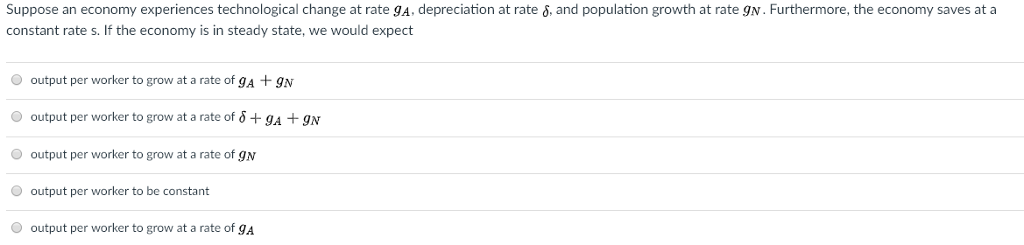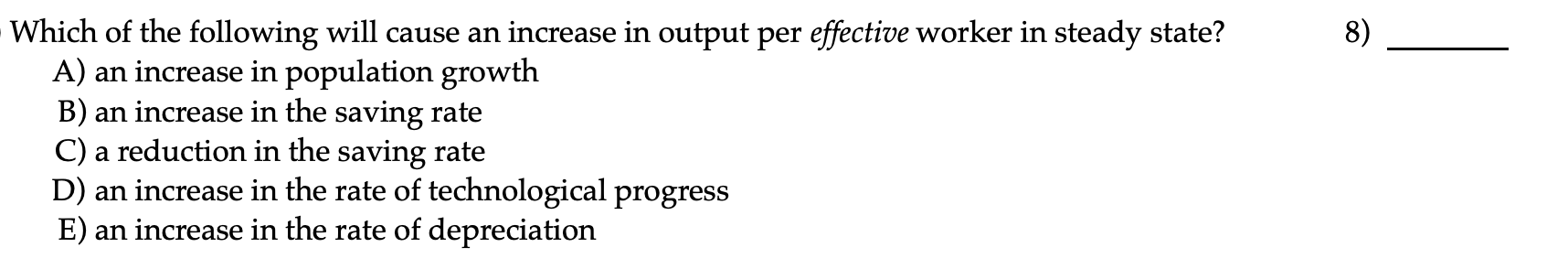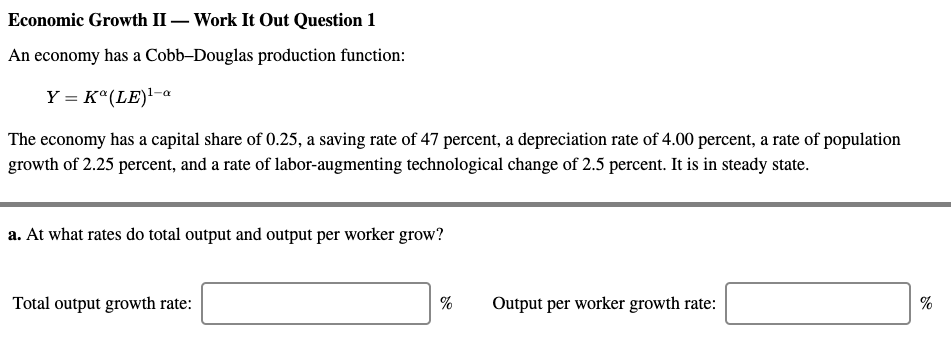# Suppose an economy experiences technological change at rate gA, depreciation at rate δ, and population growth...

Suppose an economy experiences technological change at rategA, depreciation at rateδ, and population growth at rategN. Furthermore, the economy saves at a constant rate s. If the economy is in steady state, we would expect

 output per worker to grow at a rate ofoutput per worker to be constant
 output per worker to grow at a rate ofoutput per worker to grow at a rate ofoutput per worker to grow at a rate of9A
We were unable to transcribe this image
We were unable to transcribe this image
We were unable to transcribe this image
We were unable to transcribe this image
We were unable to transcribe this image
We were unable to transcribe this image

If the economy is in steady state and there is technological growth at the rate of GA, then the output per worker will always grow at the rate of growth of technology i.e GA.

Hence the correct answer is (D).

(I hope you satisfied)

#### Earn Coin

Coins can be redeemed for fabulous gifts.

Similar Homework Help Questions
• ### Suppose an economy experiences technological change at rate gA-depreciation at rate δ, and population growth at...Suppose an economy experiences technological change at rate gA-depreciation at rate δ, and population growth at rate gN. Furthermore, the economy saves at a constant rate s. If the economy is in steady state, we would expect output per worker to grow at a rate of gA +9N O output per worker to grow at a rate of δ + 9A + gN output per worker to grow at a rate of gN O output per worker to be constant...

• ### Suppose an economy experiences technological change at rate g A, depreciation at rate g, and population...

Suppose an economy experiences technological change at rate g A, depreciation at rate g, and population growth at rate g N. Furthermore, the economy saves at a constant rate s. If the economy is in steady state, we would expect capital to grow at a rate of g N capital to grow at a rate of gA + gN capital to grow at a rate of g + gA + gN capital to grow at a rate of gA capital...

• ### Consider an economy with population growth n, depreciation rate δ and technological progress g. With the...

Consider an economy with population growth n, depreciation rate δ and technological progress g. With the aid of graphs, explain what happens to the steady-state capital per effective worker and income per effective worker in response to each the following: (a) Local events causes a shift in consumer preferences, leading to a decline in the saving rate (b) Greater access to birth control leads to a reduction in the rate of population growth

• ### An economy with a population growth rate at 2 percent and a rate of technological growrh...

An economy with a population growth rate at 2 percent and a rate of technological growrh at 3 percent is in steady state. If the capital-output ratio is 2, depreciation amounts to 10 percent of GDP, and capital income is 20 percent of GDP, then this economy would need to ......... the Golden Rule steady state. (Please, is it to decrease or increase saving rate, s, or do nothing. OR do I decrease the steady state stock of capital per...

• ### An economy has the following production function: Y = K1/2L 1/2 There is no technological growth...

An economy has the following production function: Y = K1/2L 1/2 There is no technological growth in the economy. Some more additional details known about the economy: • The savings rate (s) is equal to 0.4. • The population growth rate (n) is equal to 0.03. • Depreciation rate (δ) is at 0.07. (a) Derive the function of output per worker in terms of capital per worker. (b) Find the steady state levels of capital per worker, output per worker...

• ### Economic Growth II - Work It Out Question 1 An economy has a Cobb Douglas production...Economic Growth II - Work It Out Question 1 An economy has a Cobb Douglas production function: Y = K (LE). The economy has a capital share of 0.20, a saving rate of 50 percent, a depreciation rate of 3.50 percent, a rate of population growth of 4.00 percent, and a rate of labor augmenting technological change of 2.5 percent. It is in steady state. a. At what rates do total output and output per worker grow? Total output growth...

• ### Is that the Y/an expression (depreciation rate + gA + gN) K/ (s*AN)? then E and...Is that the Y/an expression (depreciation rate + gA + gN) K/ (s*AN)? then E and C and both work? if I'm wrong, please tell me why and what is the correct expression of Y/an, thank you so much - Which of the following will cause an increase in output per effective worker in steady state? A) an increase in population growth B) an increase in the saving rate C) a reduction in the saving rate D) an increase in...

• ### Consider an economy in a steady state with population growth rate η, a rate of capital depreciati...

Consider an economy in a steady state with population growth rate η, a rate of capital depreciation δ , and a rate of technological progress g. a)  At the steady state Δk = 0, where k equals capital per effective worker. What condition must be met for this to hold? Describe the condition in words as well as mathematical expressions. b) Describe in words what is maximized at the Golden Rule level of k. c) What mathematical condition must be...

• ### Economic Growth II — Work It Out Question 1 An economy has a Cobb-Douglas production function:...Economic Growth II — Work It Out Question 1 An economy has a Cobb-Douglas production function: Y = K (LE)-a The economy has a capital share of 0.25, a saving rate of 47 percent, a depreciation rate of 4.00 percent, a rate of population growth of 2.25 percent, and a rate of labor-augmenting technological change of 2.5 percent. It is in steady state. a. At what rates do total output and output per worker grow? Total output growth rate: %...

• ### Suppose there are two very similar countries (call them E and F). Both countries have the...

Suppose there are two very similar countries (call them E and F). Both countries have the same population and neither is experiencing population growth (that is, N is identical and constant in both countries). Both countries depreciate capital at the same rate, the both have the same savings rate, they both have the same technology, and there is no technological progress. Suppose that currently both countries are in steady state, when an earthquake destroys half of the capital stock of...

Free Homework App